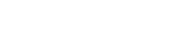# math.asinh

`FLOATmath.asinhFLOATx`

Available inall subroutines.

Computes the inverse hyperbolic sine of its argument `x`.

## Parameters

`x` - Floating point value representing the area of a hyperbolic sector.

## Return Value

Upon successful completion, this function returns the inverse hyperbolic sine of `x`.

If `x` is `math.NAN`, a NaN will be returned.

If `x` is ±0, or `math.POS_INFINITY` or `math.NEG_INFINITY`, `x` will be returned.

If `x` is subnormal, a range error occurs and `x` will be returned.

## Errors

If the `x` argument is subnormal, then fastly.error will be set to `ERANGE`.

## Example

``````declare local var.fo FLOAT;
set var.fo = math.asinh(1);``````

BETA